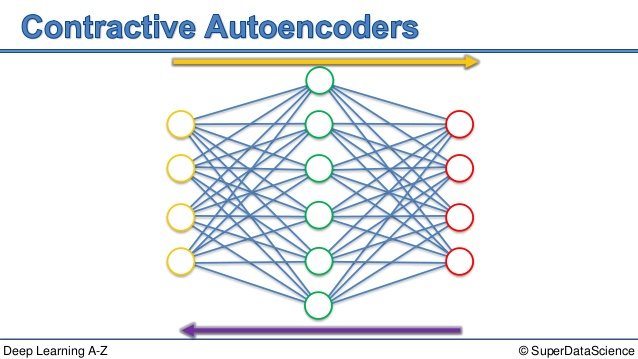/  Deep Learning Interview questions and answers   /  Explain about the Contractive autoencoders?## Explain about the Contractive autoencoders?

Ans: A contractive autoencoder is an unsupervised deep learning technique that helps a neural network encode unlabeled training data. Contractive autoencoder (CAE) objective is to have a robust learned representation which is less sensitive to small variation in the data.

Robustness of the representation for the data is done by applying a penalty term to the loss function. The penalty term is Frobenius norm of the Jacobian matrix. Frobenius norm of the Jacobian matrix for the hidden layer is calculated with respect to input. Frobenius norm of the Jacobian matrix is the sum of square of all elements.

CAE surpasses results obtained by regularizing autoencoder using weight decay or by denoising. CAE is a better choice than denoising autoencoder to learn useful feature extraction.

Penalty term generates mapping which are strongly contracting the data and hence the name contractive autoencoder.